# Percent of Change

In this lesson, you will expand your knowledge of percent problems by learning to solve problems involving percent of change. There is one simple technique to follow: To find the percent increase or the percent decrease, write the difference in amounts as the numerator of a fraction with the original amount as the denominator. Then, change the fraction to a percent.

Let us simplify that process by using a formula.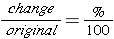Write this formula down because you will be using it often during this lesson.

### Example 1A laptop that originally costs \$795 is on sale for \$600. What is the percent decrease of the price of the laptop?

Strategy: Write a fraction to represent the decrease.

Step 1 – Write a fraction and simplify.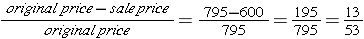Step 2 – Convert the fraction to a percent.Solution – The percent decrease in the price of the laptop is 24.5%.

### Example 2A basketball team won 15 games during the 2013-2014 season. In the 2014-2015 season, the team won 21 games. What was the percent of increase in games won?

Strategy: Write a fraction to represent the increase.

Step 1 – Write a fraction and simplify.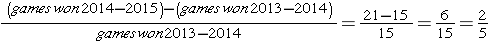Step 2 – Convert the fraction to a percent.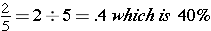Solution – The percent increase in games won is 40%.

Note: You will not have negative numbers in the differences – use absolute value for this value. Just find the difference – always subtract the smaller value from the larger.

See if you can find the percent of change for this problem.

There were 80 seventh-grade students who signed up for track tryouts last year. This year, 68 seventh-grade students signed up for tryouts. What is the percent decrease in the number of students from last year to this year? Make sure to find the answer before moving on.

The next example is presented a bit differently; however, you use the same process to solve. Take a close look at this one.

### Example 3An art gallery owner purchased a very old painting for \$5,000. Then, he sells the painting at a 315% increase in price. What is the retail price of the painting?

Strategy: Write a fraction to represent the increase.

Step 1 – Write a fraction and cross multiply.Step 2 – Solve for x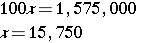This is the change – not the sale price.

Step 3 –  Add the change of the price to the original price 15,750 + 5000= 20,750

Solution – The actual retail price of the painting at 315% increase is \$20,750.00

*Check your work by plugging this information into the formula.Let’s look at one more problem similar to this one.

### Example 4In August, Jamie spent \$48 on gas for her car. In September, she spent 15% less. How much did she spend on gas for her car in September?

Strategy: Write a fraction to represent the decrease.

Step 1 – Write a fraction and cross multiply.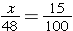Step 2 – Solve for x: 100x = 720 ; x = 7.20 This is the decrease – not the solution

Step 3 – Subtract the change of the price from the original price (it is a decrease) \$48 – \$7.20 = \$40.80

Solution – Jamie spent \$40.80 on gas for her car in September.

*Check your work by plugging this information into the formula.That is all there is to it. Now, complete the following learning activity and you are ready to move forward. Remember to have your pencil, paper, and a calculator handy when you work problems involving percents.

(source)